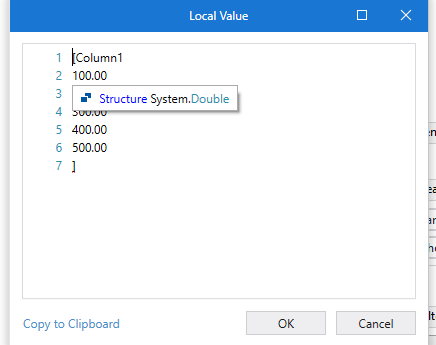# Filter dt by double precision numbers

Hey!
I am trying to filter the database by column “Column1”> 100, but unsuccessfully (changed to 100.00).
In this column, the data type is double. How do I filter out doubles? Thanks.Main.xaml (6.0 KB)
sample.xlsx (7.9 KB)

Hi,

Your data in the datatable seems recognized as String type.So, perhaps you should use other way to filter it. For example, LINQ as the following expression.

``````dt_filter = dt.AsEnumerable.Where(Function(r) Double.parse(r("Column1").ToString)>100).CopyToDataTable
``````

Regards,

1 Like

Thanks. Is it possible to convert all rows of Column1 to double then work with regular filters (like in my example)?

Hi,

Yes, If we rewrite data as Double type. For example, as the following.

Regards,

Thanks. Sorry, but is there a way to transform a query using LINQ, so there will be about 20 thousand rows in the database?

Hi,

Alright.

If your datatable has just one column, the following will work

``````dt = dt.AsEnumerable.Select(Function(r) dt.Clone.LoadDataRow({Double.Parse(r(0).ToString)},False)).CopyToDataTable
``````

If there are 2 or more columns, try Invoke Code activity with the following code.

``````dt = dt.AsEnumerable.Select(Function(r)
r(0) =  Double.Parse(r(0).ToString)
Return r
End Function).CopyToDataTable
``````

Regards,

2 Likes

Thanks a lot, it’s awesome.

Hi!
Another good question. If there are null values ​​in the column, how can they be handled? Thanks.

Hi,

Do you want to handle it: for example, as 0 or delete the row?

Regards,

Example as 0. Thanks.

Hi,

The following code handle null or illegal characters as 0.

``````dt = dt.AsEnumerable.Select(Function(r)
r(0) =  Double.Parse(r(0).ToString)
if (r(0) isnot Nothing AndAlso Double.TryParse(r(0).ToString,New Double), Double.Parse(r(0).ToString),0)
Return r
End Function).CopyToDataTable
``````

Regards,

Thank you, but why is that an error. Need to import additional libraries?

Hi,

Sorry, I had a mistake. Can you try the following?

``````dt = dt.AsEnumerable.Select(Function(r)
r(0) = If (r(0) IsNot Nothing AndAlso Double.TryParse(r(0).ToString,New Double), Double.Parse(r(0).ToString),0)
Return r
End Function).CopyToDataTable
``````

Regards,

It does not work as expected, now all values ​​have been turned to 0.

Hi,

Can you run the following sample?
If it works in your environment, can you check differentiate b/w this and yours?

Sample20210910-2.zip (14.6 KB)

Regards,

This topic was automatically closed 3 days after the last reply. New replies are no longer allowed.